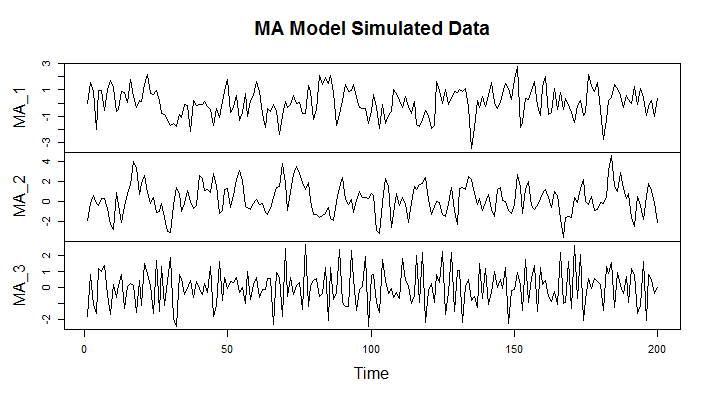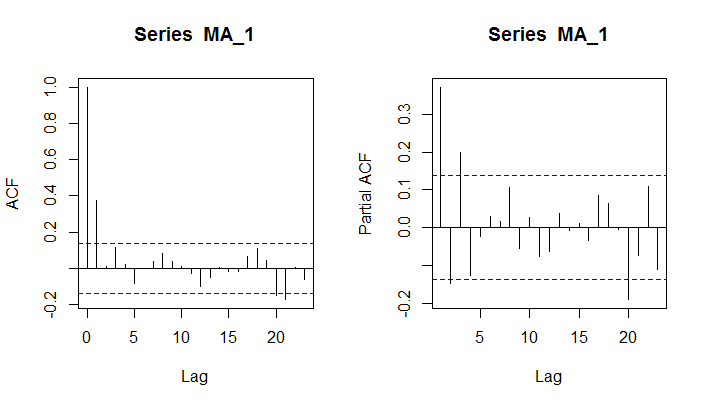# Moving Average (MA) Model in R

A Moving Average is a process where each value is a function of the noise in the past observations. These are the random error terms which follow a white noise process. The general form is MA(q), where xt depends on q past values.

Just like AR models, this also has a regression like structure, however, instead of the actual values, we are regressing each value on the noise/error in the previous observations.

We can use the arima.sim() function to simulate the MA model. For the MA model, we will specify model as list(ma = theta) , where theta is the slope parameter from the interval (-1, 1).

Below we create two sets of simulations with MA model, one with a slope of 0.5 and another with a slope of 0.8.

# Simulate AutoRegressive model with 0.5 slope
MA_1 <- arima.sim(model = list(ma = 0.5), n = 200)
# Simulate AutoRegressive model with 0.8 slope
MA_2 <- arima.sim(model = list(ma = 0.9), n = 200)
# Simulate AutoRegressive model with -0.6 slope
MA_3 <- arima.sim(model = list(ma = -0.6), n = 200)
plot.ts(cbind(MA_1 , MA_2, MA_3 ), main="MA Model Simulated Data")### ACF and PACF of Autoregressive Model

We can calculate the Autocorrelation and Partial Autocorrelation functions of the Moving Average model using the acf() and the pacf() functions.

The following are the respective ACF and PACF plots for the MA_1 series.

> acf(MA_1)
> pacf(MA_1)